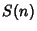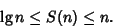## Binary Tree

A Tree with two Branches at each Fork and with one or two Leaves at the end of each Branch. (This definition corresponds to what is sometimes known as an extended'' binary tree.) The height of a binary tree is the number of levels within the Tree. For a binary tree of heightwithnodes,These extremes correspond to a balanced tree (each node except the Leaves has a left and right Child, and all Leaves are at the same level) and a degenerate tree (each node has only one outgoing Branch), respectively. For a search of data organized into a binary tree, the number of search stepsneeded to find an item is bounded byPartial balancing of an arbitrary tree into a so-called AVL binary search tree can improve search speed.

The number of binary trees withinternal nodes is the Catalan Number(Sloane's A000108), and the number of binary trees of heightis given by Sloane's A001699.

References

Lucas, J.; Roelants van Baronaigien, D.; and Ruskey, F. Generating Binary Trees by Rotations.'' J. Algorithms 15, 343-366, 1993.

Ranum, D. L. On Some Applications of Fibonacci Numbers.'' Amer. Math. Monthly 102, 640-645, 1995.

Ruskey, F. Information on Binary Trees.'' http://sue.csc.uvic.ca/~cos/inf/tree/BinaryTrees.html.

Ruskey, F. and Proskurowski, A. Generating Binary Trees by Transpositions.'' J. Algorithms 11, 68-84, 1990.

Sloane, N. J. A. Sequences A000108/M1459 and A001699/M3087 in An On-Line Version of the Encyclopedia of Integer Sequences.'' http://www.research.att.com/~njas/sequences/eisonline.html and Sloane, N. J. A. and Plouffe, S. The Encyclopedia of Integer Sequences. San Diego: Academic Press, 1995.